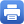Columbia Home
Mandatory MAFN Courses

### Fall

MATH GR 5010 Introduction to the Mathematics of Finance
Sec. 001

The mathematics of finance, principally the problem of pricing of derivative securities, developed using only calculus and basic probability. Topics include mathematical models for financial instruments, Brownian motion, normal and lognormal distributions, the Black-Scholes formula, and binomial models.

STAT GR 5263 Statistical Inference / Time-Series Modeling
Sec. 001 and Sec. 002

Modeling and inference for random processes, from natural sciences to finance and economics. ARMA, ARCH, GARCH and nonlinear models, parameter estimation, prediction and filtering.

STAT GR 5264 Stochastic Processes – Applications
Sec. 001 and Sec. 002

Basics of continuous-time stochastic processes. Wiener processes. Stochastic integrals. Ito’s formula, stochastic calculus. Stochastic exponentials and Girsanov’s theorem. Gaussian processes. Stochastic differential equations. Additional topics as time permits.

### Spring

STAT GR 5265 Stochastic Methods in Finance
Sec. 001 and Sec. 002

Mathematical theory and probabilistic tools for modeling and analyzing security markets are developed. Pricing options in complete and incomplete markets, equivalent martingale measures, utility maximization, term structure of interest rates.

MATH GR 5030 Numerical Methods in Finance
Sec. 001

Review of the basic numerical methods for partial differential equations, variational inequalities and free-boundary problems. Numerical methods for solving stochastic differential equations; random number generation, Monte Carlo techniques for evaluating path-integrals, numerical techniques for the valuation of American, path-dependent and barrier options.

MATH GR 5050 Practitioners’ Seminar
Sec. 001

The seminar consists of presentations and mini-courses by leading industry specialists in quantitative finance. Topics include portfolio optimization, exotic derivatives, high frequency analysis of data and numerical methods. While most talks require knowledge of mathematical methods in finance, some talks are accessible to a general audience.Print this page